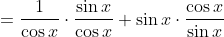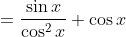Trigonometry : Apply Basic and Definitional Identities

Example Questions

Example Question #1 : Apply Basic And Definitional Identities

Which of the following trigonometric identities is INCORRECT?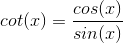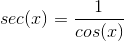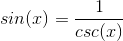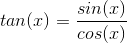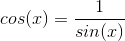Explanation:

Cosine and sine are not reciprocal functions.and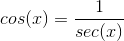Example Question #2 : Apply Basic And Definitional Identities

Using the trigonometric identities prove whether the following is valid: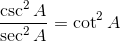Only in the range of: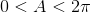Uncertain

Only in the range of: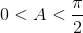False

True

True

Explanation:

We begin with the left hand side of the equation and utilize basic trigonometric identities, beginning with converting the inverse functions to their corresponding base functions: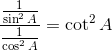Next we rewrite the fractional division in order to simplify the equation: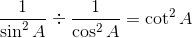In fractional division we multiply by the reciprocal as follows: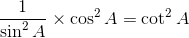If we reduce the fraction using basic identities we see that the equivalence is proven: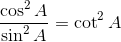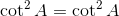Example Question #3 : Apply Basic And Definitional Identities

Which of the following identities is incorrect?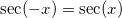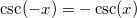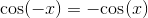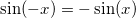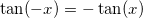Explanation:

The true identity is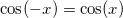because cosine is an even function.

Example Question #4 : Apply Basic And Definitional Identities

State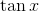in terms of sine and cosine.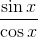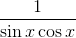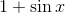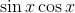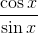Explanation:

The definition of tangent is sine divided by cosine.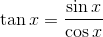Example Question #5 : Apply Basic And Definitional Identities

Simplify.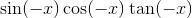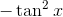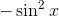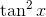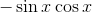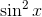Explanation:

Using these basic identities: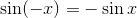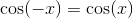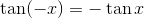we find the original expression to be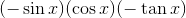which simplifies to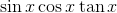.

Further simplifying: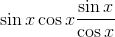The cosines cancel, giving usExample Question #6 : Apply Basic And Definitional Identities

Which of the following is the best answer for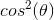?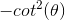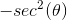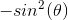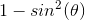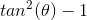Explanation:

Write the Pythagorean identity.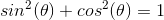Substract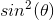from both sides.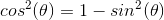Example Question #7 : Apply Basic And Definitional Identities

Express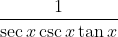in terms of only sines and cosines.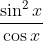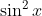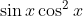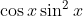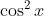Explanation:

The correct answer is. Begin by substituting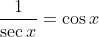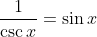, and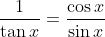. This gives us: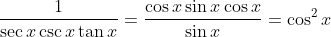.

Example Question #8 : Apply Basic And Definitional Identities

Express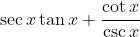in terms of only sines and cosines.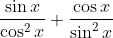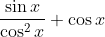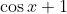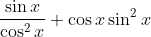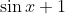Explanation:

To solve this problem, use the identities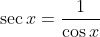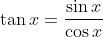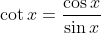, and. Then we get